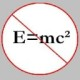You are currently browsing the tag archive for the ‘polyhedra’ tag.

A polyhedron is a solid which has a surface that consists of a number of polygonal faces. A polygon is a planar figure that is bounded by a closed path consisting of a finite sequence of straight line segments. For example, a cube or a tetrahedron is a polyhedron, while a triangle or a rectangle is a polygon.

Now, the Euler characteristic$\chi$ for polyhedra is a function (more technically, a topological invariant) defined by$\chi = V - E + F$,

where$V, , E$ and$F$ are the number of vertices, edges and faces, respectively, in a given polyhedron.

It turns out that for a simply connected polyhedron,$\chi = 2$. Therefore,$V - E + F = 2$

is Euler’s formula for a simply connected polyhedron. Prof David Eppstein has a webpage that lists no less than nineteen proofs of the above formula.

Okay, so what has Euler’s formula for polyhedra got to do with the Platonic solids? Well, using this formula we can show/prove that there are exactly five Platonic solids.

The proof below will essentially employ the common technique (in combinatorics) of counting some objects in two different ways and equating the answers in order to obtain some useful information.

So, suppose a regular polyhedron has$V$ vertices,$E$ edges and$F$ faces, each of which is an$n$-sided regular polygon. Also, suppose$m$ edges meet at each vertex.

Let the “degree of each face” be equal to the the number of edges that belong to it. Also, let the “degree of each vertex” be equal to the number of edges meeting it.

Now, since each face has$n$ edges surrounding it, the sum of the degrees of all the faces is$nF$. But, since each edge belongs to exactly two faces, each edge is counted twice in the expression$nF$. Therefore, we have$nF = 2E \quad \quad (1)$

Again, since each vertex has$m$ edges meeting it, the sum of the degrees of all the vertices is$mV$. But, since each edge meets exactly two vertices, each edge is ,again, counted twice in the expression$mV$. Therefore, we have$mV = 2E \quad \quad (2)$

Now, using$(1)$ and$(2)$ in Euler’s formula, we eliminate$V$ and$F$ to obtain$\displaystyle \frac{2E}{m} - E + \frac{2E}{n} = 2$,

which yields$\displaystyle \frac1{m} + \frac1{n} = \frac12 + \frac1{E} \quad \quad (3)$

Further, we note that a polygon must have at least three sides (else it’s not really a polygon!); therefore,$n \ge 3.$ In addition, it is easy to picture that at least three edges must meet a vertex (else a polyhedral angle at each vertex wouldn’t exist!); therefore,$m \ge 3$. But, note that$m$ and$n$ both cannot be greater than 3, for otherwise, the left hand side of equation$(3)$ cannot exceed$1/2$ whereas the right hand side of the equation exceeds$1/2$.

So, finally, we have two cases to consider.$1^{\circ}: m = 3$. Plugging this value of$m$ into equation$(3)$, we find that the possible values of$n$ are$3, 4$ or$5$. This yields$E = 6, 12$ or$30$, which correspond to the tetrahedron, cube and dodecahedron, respectively.$2^{\circ}: n = 3$. Plugging this value of$n$ into equation$(3)$, we find that the possible values of$m$ are again$3, 4$ or$5$. This yields$E = 6, 12$ or$30$, which correspond to the tetrahedron, octahedron and icosahedron, respectively.

Hence, there exist no more than five platonic solids, viz. tetrahedron, cube, octahedron, dodecahedron and icosahedron. For each of those solids, the corresponding “(vertices, edges, faces)” triples are$(4, 6, 4), , (8, 12, 6), , (6, 12, 8), , (20, 30, 12)$ and$(12, 30, 20)$, respectively.

I stole the picture below from here.

And this concludes our proof.

### Blog Stats

• 356,110 hitsJohn Favors on Solution to POW-13: Highly…Wayne J. Mann on Solution to POW-12: A graph co…erneststephen on The 54th Carnival of Math…anhtraisg on p^q + q^p is primeprof dr drd horia or… on My first postprof drd horia orasa… on My first postprof dr mircea orasa… on Inequality with lognotedscholar on Self-referential Paradoxes, In…prof dr mircea orasa… on Inequality with logprof dr mircea orasa… on Inequality with logprof dr mircea orasa… on 2010 in reviewkenji on Basic category theory, Iprof dr mircea orasa… on Solution to POW-10: Another ha…prof drd horia orasa… on Continued fraction for eprof drd horia orasa… on Inequality with log